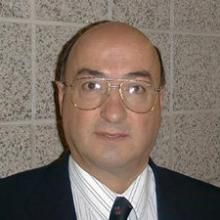# Dr. Napoleon GauthierDr. Napoleon Gauthier
BA, BSc, MSc, PhD
Office:
Sawyer Building, Room 3301
Telephone:
(613) 541-6000 ext 6332
Fax:
(613) 541-6040
E-mail:
Department of Physics

PO Box 17000, Station Forces
K7K 7B4

## Papers Published Recently

• Matrices and their Use in the Study of 3 x 3 Magic Squares, by N. Gauthier, The Mathematical Gazette, 81, 225-230 (1997).
• Identities for a Class of Sums Involving Horadam's Generalized Numbers {W_n }, by N. Gauthier, The Fibonacci Quarterly, *36*, 295-304 (1998).
• A FiboSingular Matrix?, by N. Gauthier, Advanced Problems Section, The Fibonacci Quarterly *35 *(1997), p. 91 and __*36 *(1998), p. 188, with solution by P.S. Bruckman.
• Sum Formula, by N. Gauthier, Advanced Problems Section, The Fibonacci Quarterly *35,* 187 (1997), and __*36*, 383-384* *(1998), with solution by N. Gauthier.
• Simple Quantum Calculation of the Rutherford Cross Section, by N. Gauthier, European Journal of Physics, *21*, L17-L18 (2000).
• The Convergence of a Lucas Series, by N. Gauthier, The Mathematical Gazette, *84,* 5 (2000).
• Comment on "Field Pattern of a Magnetic Dipole [Am. J. Phys. *68*, 577-579 (2000)] by J.P. McTavish", by N. Gauthier, The American Journal of Physics; *69 (3)*, 384-385 (2001).
• Letter to the Editor, Physics Education, Comment on "A Simple Experiment to Study Parabolic Surfaces [Phys. Edu, *35*, 120-123 (2000)] by M. Basta, V. Picciarelli and R.Stella", by N. Gauthier, Physics Education .
• Letter to the Editor, The Mathematical Intelligencer: "Parsing a Magic Square", by N. Gauthier, *22 (4)*, 4 (2000).
• Published in The Math. Gaz. *84*, Problem Corner, #83.H, p. 133, by N. Gauthier and J.R. Gosselin: "Given the Diophantine Equation q^n+1 -m^n > q+m^n -1=0, find all the solutions for integers."
• Letter to the Editor, The American Journal of Physics: 'Magnetic braking references', by N. Gauthier, *70.2*, 103 (2002).
• Comment on 'Using Feynman diagrams to solve the classical harmonic oscillator,' by A. Thorndike [Am. J. Phys. *68(2)*, 155-159 (2000)], by N. Gauthier, Am. J. Phys. *70(2)*, 186-187 (2002).
• Comment on a Bruckman conjecture, by N. Gauthier, The Fib. Q. *39(1)*, 90 (2001). This was followed by Bruckman's "A response to Gauthier's comments on the Bruckman conjecture", The Fib. Q. *39(5)*, 471-472 (2001).
• Magnetic braking references, by N. Gauthier, Am. J. Phys.* 70(2)*, 103 >(2002).
• A Newton-Faraday Approach to Electromagnetic Energy and Angular Momentum Storage in an Electromechanical System, American Journal of Physics, *70(10)*, 1034-1038 (2002).
• The Equivalence Principle and the Dynamics of an Accelerated Helium-Filled Balloon, by N. Gauthier, The Physics Teacher, *40(8)*, 474-475 (2002).
• Letter to the Editor, International Journal of Mathematical Education in Science and Technology; *34(2)*, 317 (2003).
• Convolving the m-th Powers of the Consecutive Integers With the General Fibonacci Sequence Using Carlitz's Weighted Stirling Polynomials of the Second Kind, by N. Gauthier; The Fibonacci Quarterly; *42*, 306-313 (2005).
• What Happens to Energy and Linear Momentum When Two Oppositely-Traveling Waves Overlap? by N. Gauthier, Am. J. Phys. *71(8)*, 787-790 (2003).
• Novel approach for solving the equation of motion of a simple harmonic oscillator, by N. Gauthier, International Journal of Mathematical Education in Science and Technology *35(3)*, 446-452 (2004).
• Jacobi's 1842 solution of the inverse-square problem, by N. Gauthier, Am. J. Phys. *72(3)*, 381-384 (2004).
• Mechanical energy and momentum of wave pulses in a dispersionless, lossless elastic medium, according to the linear theory, by N. >Gauthier and P. Rochon, Am. J. Phys. *72(9)*, 1227-1231 (2004).
• Solving the inverse-square problem with complex variables, by N. Gauthier, International Journal of Mathematical Education in Science and Technology *36*, 103-109 (2005).
• Sum of the /m/-th powers of /n/ successive terms of an arithmetic >sequence: b^m + (a+b)^m + (2a+b)^m + ... + ((n-1)+b)^m , by N. Gauthier, International Journal of Mathematical Education in Science and Technology *37 (2)*, 207-215 (2006).
• Sums of the even integral powers of the cosecant and secant, by N. Gauthier and Paul S. Bruckman, The Fibonacci Quarterly *44(3)*, 264-273 (2007).